# Mathematics - Signals And Systems - Sinusoidal and Complex Exponential Signals.

in StemSocial3 months ago[Image1]

## Introduction

Hey it's a me again @drifter1!

Today we continue with my mathematics series about Signals and Systems in order to cover Sinusoidal and Complex Exponential Signals.

So, without further ado, let's get straight into it!

## Sinusoidal Signals[Image 2]

Sinusoidal signals are periodic signals, which are based on the trigonometric functions sine (sin) and cosine (cos).

### Continuous-Time Sinusoidals

In continuous-time, the generic form of a sinusoidal signal is: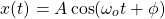where:

• A: amplitude
• ωo: angular frequency
• φ: phase shift

#### Period

The period of a sinusoidal signal is given by: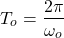#### Present Ampltiude

The signal's amplitude at present (time = to = 0) can be easily calculated using: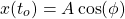because only the phase shift φ affects the present value.

#### Time Shift - Phase Shift Relationship

Time shifting a sinusoidal signal is related to phase shifting as follows: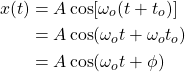Thus, in this example, the time shift by to is equal to a phase shift by φ = ωoto.

Or, the other way around, a phase shift by any φ implies a time shift by some unknown multiple of ωo.

#### Even Sinusoidal

When the phase shift is φ = 0, the sinusoidal signal A cos ωot is falling into the even category.

For a signal to be even the following must be true: x(t) = x(-t), which is of course true for the cosine function, as: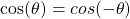#### Odd Sinusoidal

In a similar way, its also possible to prove that the sine function is odd, because: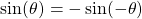Thus, the signal A sin ωot is considered an odd signal.

Its worth noting that the sine and cosine functions/signals differ by φ = -π/2. So, its easy to conclude that phase shifting by φ = -π/2, the cosine signal is also considered an odd signal: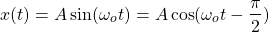### Discrete-Time Sinusoidals

In discrete-time, the sinusoidal signal is given by: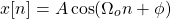where:

• A: amplitude
• Ωo: angular frequency
• φ: phase shift

#### Time Shift - Phase Shift Relationship

In the case of discrete-time, time shifting again implies a phase shift: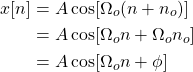So, a time shift by no samples is equal to a phase shift by Ωono.

Let's note that phase shifting now doesn't imply a time shift, as the sample rate affects the outcome of phase shifting. As a result: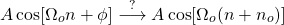#### Even-Odd Sinusoidal

Similar to continuous-time, the cosine signal is again considered an even signal, whilst the sine signal an odd signal: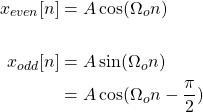#### Requirements for Periodicity

Any sinusoidal signal is considered an periodic signal in continuous-time, but in discrete-time things change slightly.

In general, in discrete-time a signal is considered periodic only when their exists a small integer N for which: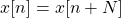In discrete-time, a sinusoidal signal: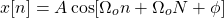is periodic when ΩoN is a multiple of , and so the following is true: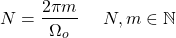The period N is the smallest natural number for which this equation is true. If none exists then the sinusoidal is aperiodic.

The following is a visualization of how the sample-rate affects the periodicity of a sinusoidal signal:[Image 3]

### Sinusoidals in Continuous- and Discrete-time at Distinct Frequencies

In addition to the issue of periodicity, continuous- and discrete-time sinusoidal also differ in other aspects.

In continuous-time, distinct values of the frequency ωo result into completely distinct signals. However, in discrete-time, values of Ωo which are separated by result into identical signals.

So, in continuous-time, if ω2 ≠ ω1 then x2(t) ≠ x1(t). But, in discrete-time, if Ω2 = Ω1 + 2πm then x2[n] ≠ x1[n].

## Exponential Signals

Exponential signals can be defined as: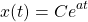where both C and a are real numbers.

### Time Shift - Scale Change Relationship

Time shifting an exponential signal implies scale change, as follows:### Complex Exponentials

Replacing C and a with complex numbers results in complex explonentials, which can be easily related to sinusoidal signals using Euler's relation.

#### Continuous-Time

In continuous-time, an complex exponential is defined as: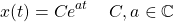where C and a tend to be defined as: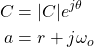which results in the following representation for complex exponentials: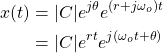Euler's relation allows us to replace the second exponential with a sum of cosine and sine: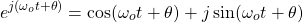And so, the final representation of complex exponentials is: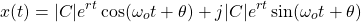where the real and imaginary parts are clearly separated.

Complex exponentials can be thought of as exponentially growing or decaying sinusoidal signals, as shown below.[Image 4]

#### Discrete-Time

In discrete-time, an complex exponential is defined as: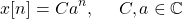where C and a are defined as: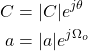therefore resulting into the following representation: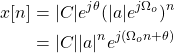Using Euler's relation, the last exponential can be replaced by sinusoidal signals, giving us the following, now final, form: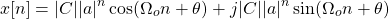## Final words | Next up

And this is actually it for today's post! Till next time!

See Ya!Keep on drifting!

Sort:Thanks for your contribution to the STEMsocial community. Feel free to join us on discord to get to know the rest of us!

Please consider supporting our funding proposal, approving our witness (@stem.witness) or delegating to the @stemsocial account (for some ROI).

Please consider using the STEMsocial app app and including @stemsocial as a beneficiary to get a stronger support.